# How to use Window Functions in SQLScriptWritten By: Irvin - 16 September 2021
(updated on: 15 March 2022)

In this article, we first define the term window function and describe how they work. Then we analyze the syntax elements of this function group and combine the shown aspects in a case study.

We use a homogeneous data set and always work with the example of the summation function.

In the appendix you will find a selection of other useful window functions, as well as a download of all tables, datasets and coding examples used in this article, so you can build on them in your own system.

Window functions are a part of all modern SQL dialects, including SQLScript.

As the name suggests, this is a command that generates a virtual "window", that is, a partition, within the SELECT command. This window represents a subset of the entire SELECT. Based on the selected function, a corresponding aggregation, for example summation, is performed within this subset.

A brief example serves as an illustration: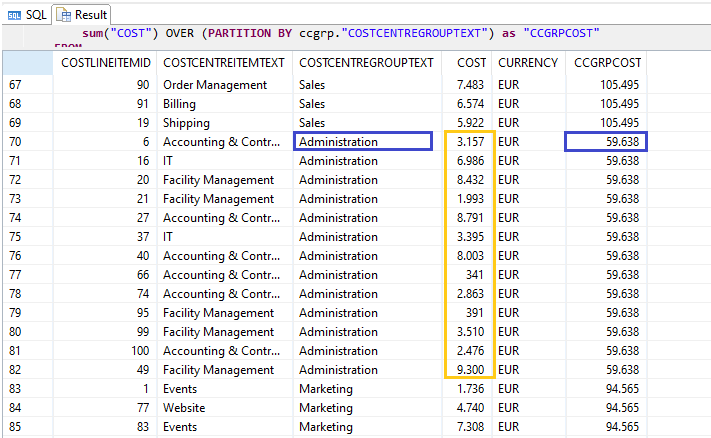Individual postings of costs with associated cost centers and cost center groups.

The result of the window function is displayed as a column with the name "CCGRPCOST". For that, the sum of all costs (marked yellow) within a cost center group (marked blue) is summed up.

With a conventional summation function, only one row would be in the output, whereas with a window function, all rows are in the output, which allows greater flexibility in the construction of SQL queries.

## Structure of the syntax

To clarify the syntax, the above example is continued: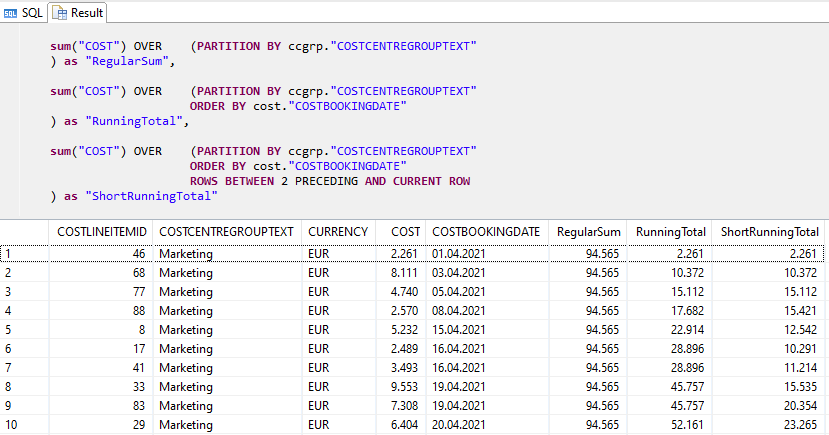Syntax examples of window functions with increasing complexity

BASICS: AGGREGATION() OVER(PARTITION BY ...)

First, the aggregation function is defined and, if necessary, parameters are passed. In this case it is sum("COST"), i.e. the sum of the column "COST".

The next command is OVER(PARTITION BY ... ) . This defines the windows on which the aggregation function is applied. Thus, here we define the column "COSTCENTREGROUPTEXT" as the window. Accordingly, the sum is calculated separately for each unique value of this column.

Although a regular sum can already be formed with the above syntax, another syntax element must be added to calculate a running sum:

ORDER BY … [ASC | DESC]

Similar to the WHERE clause of a simple SQL query, the ORDER BY keyword can also be used in a window function. This defines the sorting within the window, which has an effect on the calculation. In the case of the summation function, this generates a running total.

ROWS BETWEEN ... PRECEDING AND ... FOLLOWING

Another element is the ROWS keyword. By adapting the arguments of this keyword, even more complex circumstances can be modeled and calculated. Accordingly, by using ROWS BETWEEN 2 PRECEDING AND CURRENT ROW, the sum of the two previous lines plus the current line is formed.## Case study: Cost analysis

Finally, we illuminate the concepts presented by means of a concrete use case.

For this, we consider an initial problem and the development of a suitable solution.

We work with the same dataset as with the previous examples.

Scenario:

In the given month, more costs were incurred than the budget actually allows. In an attempt to identify potential savings, the controlling department would like to analyze which are the largest cost items in each area. In doing so, a smaller department with a smaller budget should not be unduly disadvantaged, which is why the cost items are set in relation to the total costs in the department.

In the HANA SQL Data Warehouse used, there are three tables that contain relevant data: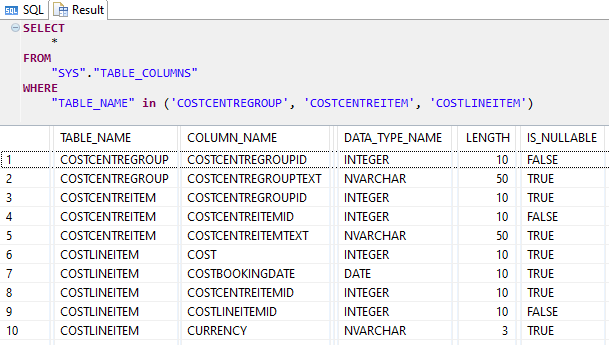First, we build a prototype in the form of an SQL query and join the above tables based on their common foreign key and primary key pairs. We assign meaningful aliases and limit the selection in the SELECT statement to relevant columns.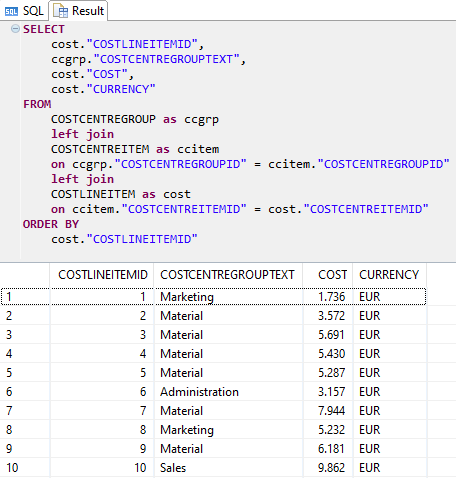Now we form the actual KPI.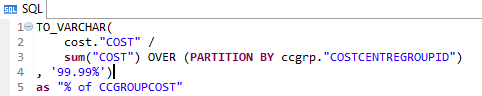The 1st and 4th lines are used to format the value as a percentage with two decimal places and the 5th line renames the column to make the output more readable.

For the value itself, we use the already given column cost."COST" as the numerator in the 2nd line and divide it by the denominator in the 3rd line.

The denominator in the 3rd line is realized by means of a window function, which calculates the sum of the cost."COST" column per ccgrp."COSTCENTREGROUPID", i.e. the sum of the costs per cost center group.

Ultimately, this gives us a percentage value that shows us the share of the respective costs in relation to the total costs of the associated cost center group.

Additionally we sort the output using the ORDER BY statement at the bottom and get the following result: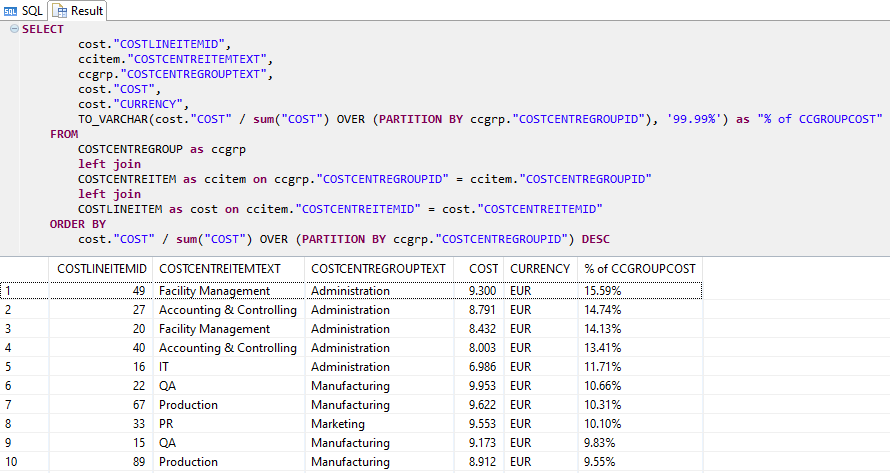Through this analysis, we were able to show that the “COSTLINEITEMID”, i.e. the cost item, with the ID '49' should be considered as the priority for saving measures.

Decision makers are happy with the analysis, which is why we provide our SQL code as a Calculation View. This makes it available as a reusable object for analogous, future analyses and can be easily integrated, visualized and shared in a front-end tool like Tableau.

## Appendix

A variety of window functions are available to enable different types of aggregations. A selection of useful variants from our consulting practice can be found below: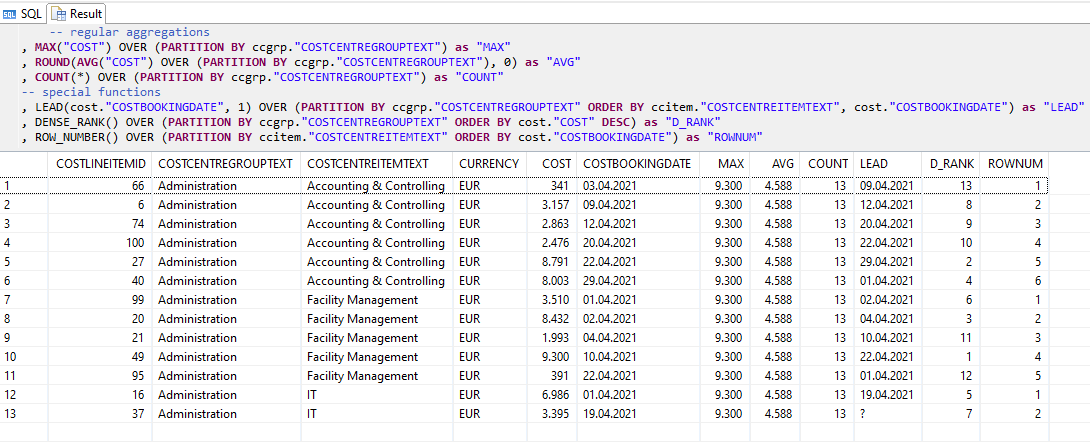You can download the tables, data sets and coding examples via the following

Do you have questions about SAP HANA SQLScript? Are you trying to build up the necessary know-how in your department or do you need support with a specific question? We are happy to help you. Request a non-binding consulting offer today!Topics: SAP BW/4HANA, SAP HANA SQL